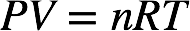# Problem: A gas sample at STP contains 1.26 g oxygen and 1.76 g nitrogen.What is the volume of the gas sample?

###### FREE Expert Solution

The ideal gas law is as follows:At conditions of standard temperature and pressure (STP), temperature = 273.15 K and pressure = 1 atm.

We first need to determine the number of moles of O2 and N2. The molar mass of O2 is 2(16.00 g/mol O) = 32.00 g/mol and the molar mass of N2 is 2(14.01 g/mol N) = 28.02 g/mol.###### Problem Details

A gas sample at STP contains 1.26 g oxygen and 1.76 g nitrogen.

What is the volume of the gas sample?